(3)       Hubble constant

We take the center  O  in the universe as reference point. Thus the velocity of a celestial body relative to the center is the absolute velocity   V´    and the distance from the center is denoted by  r´.

After the universe starts to expand on repulsion, all celestial bodies move in center repulsion field. According to the work-energy principle, we have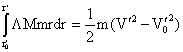since Initial velocity  V0´  may be neglected, we getor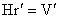here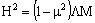(8)

when  m → 0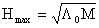(9)

We note here that L= constant.

The speed of a celestial body relative to the earth is  V ,  its distance from the earth is  r.

It can be proved that celestial bodies A, B, C, D, E and F in Fig.2 have Hubble relation with respect to the earth P. With B as instance, since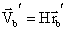and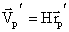we see that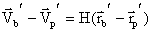and therefore

V = H r

this is just Hubble law.

Wherever you standing on any one of galaxies, you will always find the same Hubble relation This is observation effect. This is also proof for the repulsion.

Fig.4  Hubble law